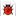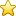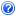# 2018版高中数学 第一章 解三角形 1.1.1 正弦定理同步精选测试 新人教B版必修5.doc 5页

## 本文档一共被下载：次 ,您可全文免费在线阅读后下载本文档。

### 下载提示

1.本站不保证该用户上传的文档完整性，不预览、不比对内容而直接下载产生的反悔问题本站不予受理。
2.该文档所得收入(下载+内容+预览三)归上传者、原创者。
3.登录后可充值，立即自动返金币，充值渠道很便利文档纠错收藏文档下载帮助

• 内容提供方 zsmfjy（上传创作收益人）
• 发布时间：2018-11-18
• 需要金币100(10金币=人民币1元)
• 浏览人气• 下载次数• 收藏次数• 文件大小：55 KB

2018版高中数学 第一章 解三角形 1.1.1 正弦定理同步精选测试 新人教B版必修5

PAGE PAGE 1同步精选测试　正弦定理(建议用时：45分钟)[基础测试]一、选择题1.在△ABC中，a＝4，∠A＝45°，∠B＝60°，则边b的值为(　　)A.eq \r(3)＋1 B.2eq \r(3)＋1C.2eq \r(6) D.2＋2eq \r(3)【解析】　由已知及正弦定理，得eq \f(4,sin 45°)＝eq \f(b,sin 60°)，∴b＝eq \f(4sin 60°,sin 45°)＝eq \f(4×\f(\r(3),2),\f(\r(2),2))＝2eq \r(6).【答案】　C2.在△ABC中，若a＝2，b＝2eq \r(3)，∠A＝30°，则∠B＝(　　)A.60° B.60°或120°C.30° D.30°或150°【解析】　由eq \f(a,sin A)＝eq \f(b,sin B)，得sin B＝eq \f(bsin A,a)＝eq \f(2\r(3)sin 30°,2)＝eq \f(\r(3),2).因为b＞a，所以∠B＞∠A，所以∠B＝60°或∠B＝120°.【答案】　B3.若三角形三个内角之比为1∶2∶3，则这个三角形三边之比是(　　) 【导学号A.1∶2∶3 B.1∶eq \r(3)∶2C.2∶eq \r(3)∶1 D.eq \r(3)∶1∶2【解析】　设三角形内角A，B，C分别为x,2x,3x，则x＋2x＋3x＝180°，∴x＝30°.由正弦定理eq \f(a,sin A)＝eq \f(b,sin B)＝eq \f(c,sin C)，可知a∶b∶c＝sin A∶sin B∶sin C，∴a∶b∶c＝sin 30°∶sin 60°∶sin 90°＝eq \f(1,2)∶eq \f(\r(3),2)∶1＝1∶eq \r(3)∶2.【答案】　B4.在△ABC中，若3b＝2eq \r(3)asin B，cos A＝cos C，则△ABC形状为(　　)A.直角三角形 B.等腰三角形C.等边三角形 D.等腰直角三角形【解析】　由正弦定理知b＝2R·sin B，a＝2R·sin A，则3b＝2eq \r(3)a3sin B＝2eq \r(3)sin A·sin B.∵0°<∠B<180°，∴sin B≠0，∴sin A＝eq \f(\r(3),2)，∴∠A＝60°或120°，又cos A＝cos C，∴∠A＝∠C，∴∠A＝60°，∴△ABC为等边三角形.【答案】　C二、填空题5.在△ABC中，∠B＝45°，∠C＝60°，c＝1，则最短边的边长等于________. 【导学号【解析】　由三角形内角和定理知：A＝75°，由边角关系知∠B所对的边b为最小边，由正弦定理eq \f(b,sin B)＝eq \f(c,sin C)得b＝eq \f(csin B,sin C)＝eq \f(1×\f(\r(2),2),\f(\r(3),2))＝eq \f(\r(6),3).【答案】　eq \f(\r(6),3)6.设△ABC的内角A，B，C的对边分别为a，b，c.若a＝eq \r(3)，sin B＝eq \f(1,2)，∠C＝eq \f(π,6)，则b＝________.【解析】　在△ABC中，∵sin B＝eq \f(1,2)，0<∠B<π，∴∠B＝eq \f(π,6)或∠B＝eq \f(5,6)π.又∵∠B＋∠C<π，∠C＝eq \f(π,6)，∴∠B＝eq \f(π,6)，∴∠A＝π－eq \f(π,6)－eq \f(π,6)＝eq \f(2,3)π.∵eq \f(a,sin A)＝eq \f(b,sin B)，∴b＝eq \f(asin B,sin A)＝1.【答案】　17.在△ABC中，若b＝5，∠B＝eq \f(π,4)，tan A＝2，则a＝________.【解析】　由tan A＝2，得sin A＝2cos A.又由sin2A＋cos2A＝1，得sin A＝eq \f(2\r(5),5).因为b＝5，∠B＝eq \f(π,4)，根据eq \f(a,sin A)＝eq \f(b,sin B)，得a＝eq \f(bsin A,sin B)＝eq \f(2\r(5),\f(\r(2),2))＝2eq \r(10).【答案】　2eq \r(10)三、解答题8.在△ABC中，已知eq \f(a,cos A)＝eq \f(b,cos B)＝eq \f(c,cos C)，试判断△ABC的形状. 【导学号【解】　令eq \f(a,sin A)＝k，由正弦定理得a＝ksin A，b＝ksin B，c＝ksin C.代入已知条件，得eq \f(sin A,cos A)＝eq \f(sin B,cos B)＝eq \f(sin C,cos C)，

“原创力文档”前称为“文档投稿赚钱网”，本站为“文档C2C交易模式”，即用户上传的文档直接卖给(下载)用户，本站只是中间服务平台，本站所有文档下载所得的收益归上传人(含作者)所有【成交的100%(原创)】。原创力文档是网络服务平台方，若您的权利被侵害，侵权客服QQ：3005833200 电话：19940600175 欢迎举报，上传者QQ群:784321556